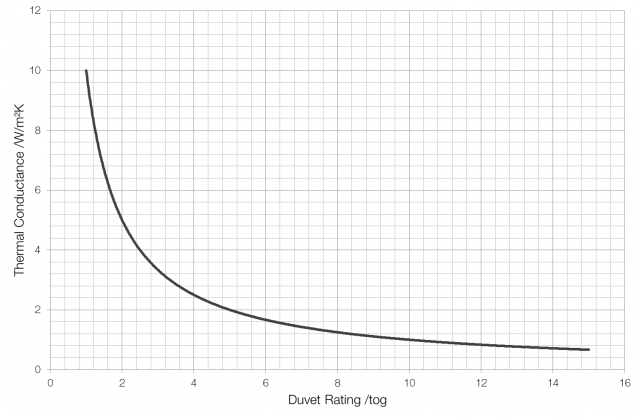Tog

Duvets are often rated by their “tog” rating. But what is tog?

Tog is a measure of a duvet’s thermal resistance. It measures the extent to which the duvet resists the transfer of thermal energy through it. One tog is equal to one-tenth of a metre squared kelvin per watt or 0.1 m²K/W. Thermal resistance can be a bit difficult to understand, but the reciprocal of thermal resistance, the thermal conductance, is a bit easier to grasp.

A one tog duvet would have a thermal resistance of 0.1 m²K/W and a thermal conductance of 10 W/m²K, a two tog duvet would have a thermal resistance of 0.2 m²K/W and a thermal conductance of 5 W/m²K, and so on.A lightweight summer duvet* has a tog rating of about four, so its thermal conductance is 2.5 W/m²K. This means that 2.5 watts of thermal energy will move through each square metre of the duvet for every one kelvin difference in temperature between the sides of the duvet.

Whilst sleeping the average person puts out about seventy watts of heat. Some of this heat will be radiated into the mattress, and some will leak out around the head and neck and edges of the duvet, but it’s not unreasonable to think that around fifty watts is going into the air surrounding the body underneath the duvet.

To maintain a constant temperature underneath the duvet the amount of heat lost must be equal to the amount of heat output by the body. If an eight tog (1.25 W/m²K) autumn duvet has an area of three square metres then this break-even point will be reached when the difference in temperature between the two sides is about thirteen degrees (50 ÷ (3 × 1.25)). Given a skin temperature of 35°C this duvet will therefore keep you at a constant temperature in a room at a temperature of 22°C. If the room is colder than 22°C then the air underneath the duvet will gradually cool down and the body will increase its rate of heat production to compensate. If the room is hotter than 22°C then the air around the body will continue to increase in temperature (until it reaches the same temperature as the body) making you uncomfortably hot and will probably cause you to throw off the duvet or stick your leg out from underneath the covers to increase the rate of heat loss.

If the duvet in the example above is replaced with a four tog summer duvet with a conductance of 2.5 W/m²K then the room would have to be a scorching 29°C, but it’s unlikely that in this situation you would want a constant temperature – you’d want to remain cool overnight. If it was replaced with a twelve tog winter duvet (conductance = 0.83 W/m²K) then the room could go down to 15°C before a net heat loss occurred.

All the calculations above are based on some unreasonable assumptions, the most obvious one being that heat is not lost throughout the whole three square metre surface of the duvet. If a person is “using” only half this area then the numbers involved change to reflect more realistic values: for a winter duvet the temperature difference required can be greater and for a summer duvet it can be smaller. The calculations also ignore the effect of any heat radiated into the mattress below the person and the insulating effect that this mattress would have.

* John Lewis classifies summer duvets as those rated at between three and four-and-a-half tog, spring/autumn duvets as those between seven and ten-and-a-half tog and winter duvets as those between twelve and thirteen-and-a-half tog.